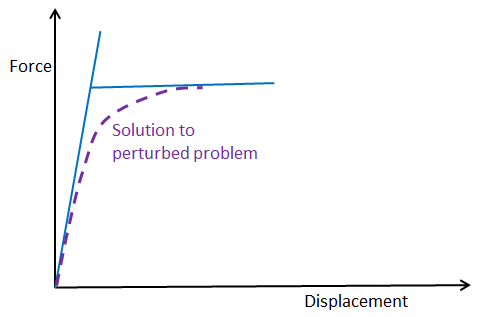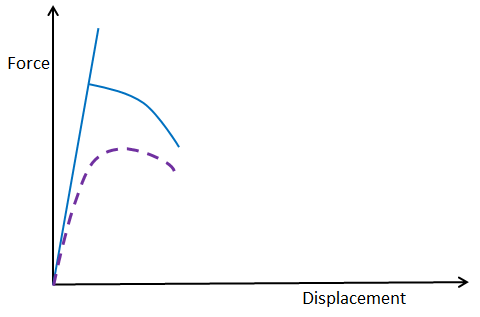# 屈曲，当结构突然坍塌时

2014年 3月 7日

### 什么是屈曲？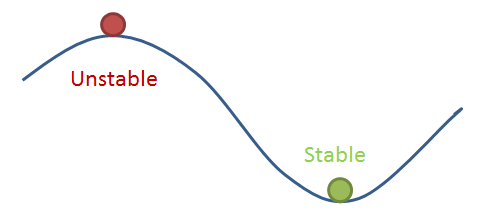### 线性屈曲分析

1. 稳态研究步骤，计算所施加载荷的应力状态。
2. 线性屈曲研究步骤。这是一个特征值解，应力状态被用来确定临界荷载因子

• 对于一些结构，由于缺陷敏感性，使用这种方法获得的理论屈曲荷载可能明显高于实际遇到的载荷。这对于薄壳尤其重要。
• 有些结构甚至在屈曲之前就表现出明显的非线性。原因可能是结构既是几何非线性又是材料非线性。
• 千万不要在屈曲分析中使用对称条件，即使结构和荷载是对称的，屈曲形状也可能不是对称的。\mathbf K = \mathbf K_L + \mathbf K_{NL}(\mathbf P)

\mathbf K = \mathbf K_L + \lambda \mathbf K_{NL}(\mathbf P_0)

( \mathbf K_L + \lambda \mathbf K_
{NL}(\mathbf P_0))\mathbf u = \mathbf 0

( (\mathbf K_L+\mathbf K_
{NL}(\mathbf P_0))+\lambda \mathbf K_{NL}
(\mathbf P_0))\mathbf u = ( \mathbf K_L+(\lambda +1) \mathbf K_
{NL}(\mathbf P_0))\mathbf u =\mathbf 0

term\lambda+1 中额外的’1’被自动补偿了，所以在两种情况下计算的载荷因子是一样。最好的法则是在预载研究步骤和屈曲研究步骤中都使用相同的几何非线性设置。

### 固定载荷和可变载荷

( \mathbf K_L + \mathbf K_{NL}
(\mathbf Q) + \lambda \mathbf K_{NL}
(\mathbf P_0))\mathbf u = \mathbf 0

1. 将它作为后屈曲分析运行，一组荷载固定，另一组荷载增加。这很简单直接，但从计算的角度来看，这种大规模的计算是不必要的。
2. 使用下文介绍的修改后的线性屈曲研究。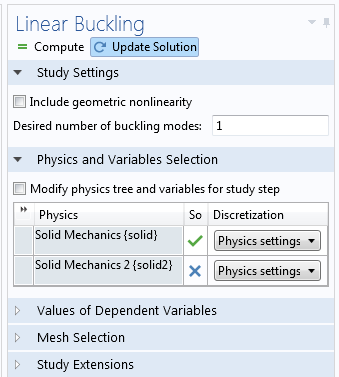-\boldsymbol\sigma^Q:\text
{test}(\mathbf E-\boldsymbol \epsilon)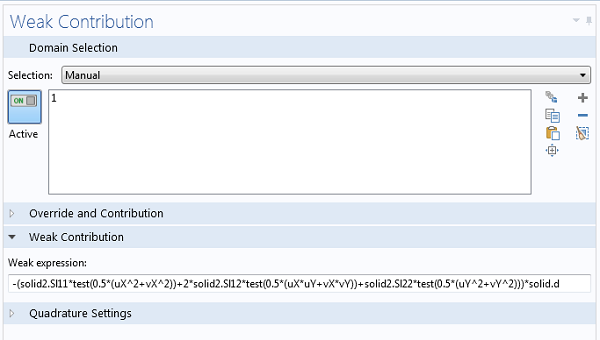### 后屈曲分析

1. 该结构在临界载荷之前也具有明显的非线性，因此线性分析并不适用。
2. 需要研究缺陷敏感性。
3. 有意在部件的后屈曲状态下进行操作。

#### 突弹跳变问题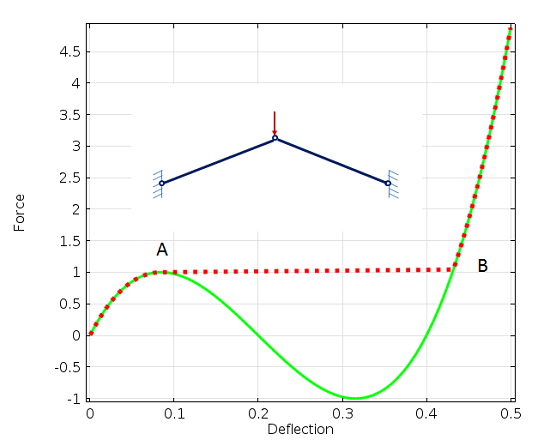• 它或多或少仅限于外部载荷为单点载荷的情况。
• 指定的位移必须是单调增加。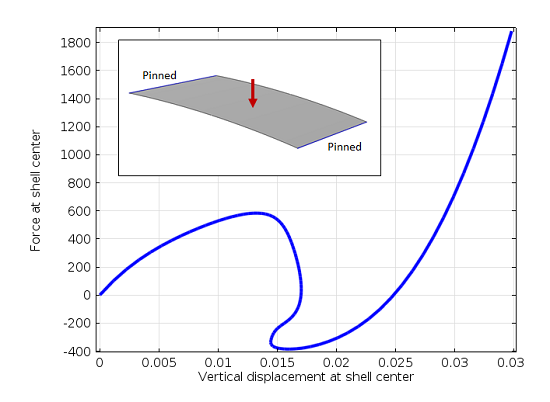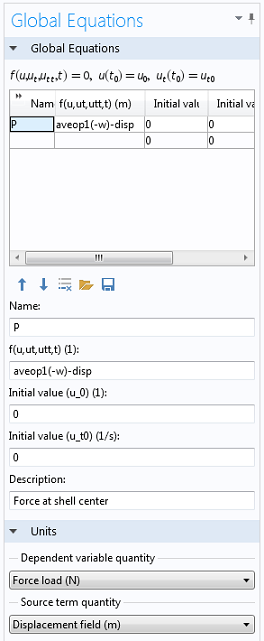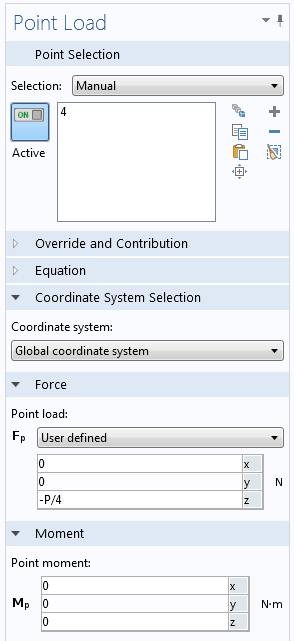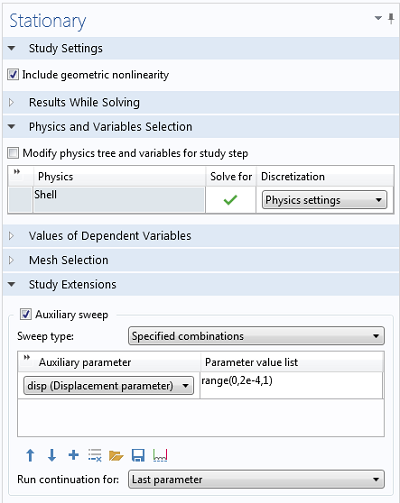#### 缺陷